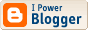## PL/SQL Procedure Call Overhead Re-visited

Zsolt Lajosfalvi wrote such an interesting comment in response to my earlier post about PL/SQL Procedure Call Overhead that I felt it deserved its own space.

What follows is exclusively the work of Zsolt. My results from verifying his tests follow at the end.

Zsolt:

In my experience the overhead of PLSQL procedure invocations is so small that you'll most probably never have to think about it. In 99.99% of all cases the "real" overhead comes from the way you do things inside the procedure(s) and not from the procedure invocation itself. So putting as much code into a single procedure as you can won't help. :-)

Let's take a look at a very basic example.
Run this:

DECLARE
nLoops CONSTANT NUMBER := 10000000;
nStart NUMBER;
PROCEDURE calculate IS
BEGIN
NULL;
END calculate;
BEGIN
dbms_output.enable(buffer_size => 10000);

nStart := dbms_utility.get_time();
FOR i IN 1..nLoops LOOP
calculate();
END LOOP;
dbms_output.put_line('time: ' || ROUND((dbms_utility.get_time() - nStart)/100, 3) || ' s');

nStart := dbms_utility.get_time();
FOR i IN 1..nLoops LOOP
NULL;
END LOOP;
dbms_output.put_line('time: ' || ROUND((dbms_utility.get_time() - nStart)/100, 3) || ' s');
END;
/

On my server the output was:
time: 4.33 s
time: 1.47 s

I ran it around 10 times and got quite similiar results with a very small deviation. This shows that even in a magnitude of 10 million invocations, the overhead is minimal (~3s which is 66% in this case). Of course this is nothing like a real world situation. :-)

Let's do some work in the procedure. What if we pass in a parameter and return a value (make it a function)?

DECLARE
nLoops CONSTANT NUMBER := 10000000;
nStart NUMBER;
nTemp NUMBER;
FUNCTION calculate(nParam IN NUMBER) RETURN NUMBER IS
BEGIN
RETURN nParam + 1;
END calculate;
BEGIN
dbms_output.enable(buffer_size => 10000);

nTemp := 0;
nStart := dbms_utility.get_time();
FOR i IN 1..nLoops LOOP
nTemp := calculate(nTemp);
END LOOP;
dbms_output.put_line('time: ' || ROUND((dbms_utility.get_time() - nStart)/100, 3) || ' s');

nTemp := 0;
nStart := dbms_utility.get_time();
FOR i IN 1..nLoops LOOP
nTemp := nTemp + 1;
END LOOP;
dbms_output.put_line('time: ' || ROUND((dbms_utility.get_time() - nStart)/100, 3) || ' s');
END;
/

The output is:
time: 12.24 s
time: 3.75 s

The overhead seems to be quite "big" compared to the previous test (~8.5s, which is ~70% in this case), however this is still as much unrealistic as the previous one was. :-) Generally you could say that the more work you do inside the function call (or procedure), the less percentage the overhead will be.

Let's do the test again with a bit more work inside the function ...

DECLARE
nLoops CONSTANT NUMBER := 10000000;
nStart NUMBER;
nTemp NUMBER;
FUNCTION calculate(nParam IN NUMBER) RETURN NUMBER IS
BEGIN
RETURN POWER(nParam + 1, 2) - POWER(nParam, 2);
END calculate;
BEGIN
dbms_output.enable(buffer_size => 10000);

nTemp := 0;
nStart := dbms_utility.get_time();
FOR i IN 1..nLoops LOOP
nTemp := calculate(nTemp);
END LOOP;
dbms_output.put_line('time: ' || ROUND((dbms_utility.get_time() - nStart)/100, 3) || ' s');

nTemp := 0;
nStart := dbms_utility.get_time();
FOR i IN 1..nLoops LOOP
nTemp := POWER(nTemp + 1, 2) - POWER(nTemp, 2);
END LOOP;
dbms_output.put_line('time: ' || ROUND((dbms_utility.get_time() - nStart)/100, 3) || ' s');
END;
/

time: 47.28 s
time: 31.1 s

Now the overhead is ~16s which is ~34% of the total running time.

All of the above tests use very fast, builtin arithemtical functions. Let's take an example that is a lot more realistic ... eg. we generate some output to a browser. The procedures in the SYS.htp package do a lot more (and versatile) work than any of the above examples. I've split this test into two parts to avoid misleading results due to the internal workings of the SYS.htp package. I've also decreased the loop count so the server won't run out of memory (since SYS.htp maintains an internal buffer of all the strings the you send to the output). :-)

DECLARE
nLoops CONSTANT NUMBER := 1000000;
nStart NUMBER;
sTemp VARCHAR2(200);
PROCEDURE calculate(sParam IN VARCHAR2) IS
BEGIN
htp.p(sParam);
END calculate;
BEGIN
dbms_output.enable(buffer_size => 10000);

htp.init();
nStart := dbms_utility.get_time();
FOR i IN 1..nLoops LOOP
calculate(sTemp);
END LOOP;
dbms_output.put_line('time: ' || ROUND((dbms_utility.get_time() - nStart)/100, 3) || ' s');

htp.init();
END;
/

And the procedure-free version ...

DECLARE
nLoops CONSTANT NUMBER := 1000000;
nStart NUMBER;
sTemp VARCHAR2(200);
PROCEDURE calculate(sParam IN VARCHAR2) IS
BEGIN
htp.p(sParam);
END calculate;
BEGIN
dbms_output.enable(buffer_size => 10000);

htp.init();
nStart := dbms_utility.get_time();
FOR i IN 1..nLoops LOOP
htp.p(sTemp);
END LOOP;
dbms_output.put_line('time: ' || ROUND((dbms_utility.get_time() - nStart)/100, 3) || ' s');

htp.init();
END;
/

You should run them in two separate sessions, otherwise you can easily end up with false results due to the package state of SYS.htp.

My results were:
time: 23.7 s
time: 23.27 s

You can see that the overhead is less than 0.5s, which makes up less than 2% of the total running time.

After all these tests proove only one thing: the overhead seriously depends on the number of parameters, the type of parameters, etc. However in real-world situations you should rarely worry about overhead of procedure invocations. :-)

Robert:
My results were very similar to Zsolt's:

anonymous block completed
time: 11.28 s
time: 4.26 s

anonymous block completed
time: 51.18 s
time: 43.24 s

anonymous block completed
time: 20.37 s

anonymous block completed
time: 19.53 s